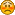Hello Guest

# [Solved] normals in low poly quads Terrain

• 3 Replies
• 1411 Views####Andrew Alfazy

•• 36##### [Solved] normals in low poly quads Terrain
« on: January 14, 2018, 07:31:02 »
Hello,
Need help with normals I'm Trying to make low poly quads Terrain (the normals for all 4 Vertices should be 1) so is this method good?
Code: [Select]
`private static Vector3f getNormal(Vector3f v1, Vector3f v2, Vector3f v3, Vector3f v4) { Vector3f a = new Vector3f(), b, n1, n2, n3, n4, n; b = a; n = a; // N1 v1.sub(v2, a); v1.sub(v4, b); n1 = a.cross(b); // N2 v2.sub(v3, a); v2.sub(v1, b); n2 = a.cross(b); // N3 v3.sub(v4, a); v3.sub(v2, b); n3 = a.cross(b); // N4 v4.sub(v1, a); v4.sub(v3, b); n4 = a.cross(b); // N n1.half(n2, a); n3.half(n4, b); a.half(b, n); return n; }`NOTE: I'm using JOML
thank you and sorry for my bad English« Last Edit: January 14, 2018, 12:23:31 by Andrew Alfazy »####Andrew Alfazy

•• 36##### Re: normals in low poly quads Terrain
« Reply #1 on: January 14, 2018, 09:33:01 »
I found the problem but I can't solve it.
the problem in the calculation how can I calculate normals for quad?
note = distance between point (x or z) and other is 10 the only y is changing.####KaiHH

•• 252##### Re: normals in low poly quads Terrain
« Reply #2 on: January 14, 2018, 11:07:48 »
If it is really a quad, that is, all vertices lie on the same plane, then you just need to take any triangle of the quad and compute the normal of it with a single cross product like so:
Code: [Select]
`// v2 - v1Vector3f v12 = v2.sub(v1, new Vector3f());// v3 - v2Vector3f v23 = v3.sub(v2, new Vector3f());// (v2 - v1) x (v3 - v2)return v12.cross(v23).normalize();`While conceptually correct (all cross products on the spanning vectors of a quad's vertices should be the same), your code suffers from aliasing between the 'nX' variables, 'a', 'b' and 'n' (i.e. those are all the same object).
« Last Edit: January 14, 2018, 11:58:09 by KaiHH »####Andrew Alfazy

•• 36##### Re: [Solved] normals in low poly quads Terrain
« Reply #3 on: January 14, 2018, 12:39:22 »
thanks now I can see how to solve itTIP:Never try to make low poly quads terrain Triangles better
« Last Edit: January 19, 2018, 08:20:55 by Andrew Alfazy »